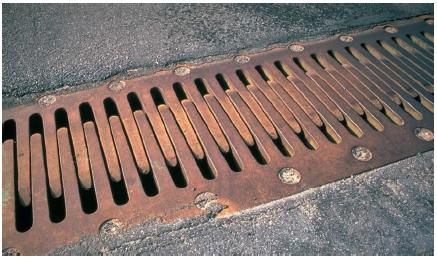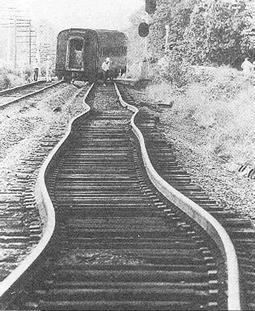# PHY2049 LLUIAL10

https://www.geogebra.org/m/JBhbf6Ke

### Objectives I• To describe thermal expansion and its applications• To investigate the proportionality relationship between length, L and Temperature, T
• How changes in temperature influence/determine changes in an object's length

### Safety precautions

• Be careful when handling the copper/aluminum rods ; they are very HOT

### Procedure I:

Watch this video to learn how to use the interactive animation and gather experimental data; Use this interactive animation link; Credits, Author: Luciano Troilo

1. Turn Heat On
2. Click on the Read Temp button 5 times, when the change in length indicator reaches approximately,
10, 20, 30,40, 50 micrometers...
3. Record the change in length from the analog dial (arc of the circle);
4. Record the T, temperature; it's shown in degrees Celsius;
5. Use the graphing script link and plot the changes, ΔL, ΔT such that the slope of the graphs represents αexpansion.
6. Identify the material, the rod is made of, by using this link and searching for a material that has αexpansion=slopefrom graph

### Theory

1. The relationship between ΔT and ΔL:

1)  :   ΔL=α⋅Linitial⋅ΔT
Consider the initial length of the rod inside the device/sleeve
Linitial= 7.8 cm

1. The slope-intercept form for the equation of a straight line is

2)  :   y= mx +b
Comparing it with the equation 1 above →:

1. ΔL is the variable on the y-axis expressed in m
2. Linitial⋅ΔT is the variable on the x-axis expressed in units of m⋅0C
• α, the linear expansion coefficient is the slope of the line, m;
• b, the y-intercept =0.

### Graphing - vizualizingΔT,ΔLrelationship

1. Plot a graph using this link !
Plot the Fahrenheit temperature on the y axis and the Celsius temperature scale on the x axis.